## Example Questions

← Previous 1

### Example Question #91 : Fractions

Flour, eggs, sugar, and chocolate chips are mixed by weight in the ratio of 12 : 5 : 3 : 5, respectively. How many pounds of chocolate chips are there in 75 pounds of the mixture?

5

25

15

18

15

Explanation:

First, add up the four parts of the ratio. This equals 25 parts. These 25 parts make up the 75 pound mixture, which means the 75 pound mixture is composed of 3 times the 25 parts (25x = 75 so x = 3).

This allows you to know that the number of pounds of chocolate chips is 3 times the ratio, i.e. 3 * 5 = 15. The mixture includes 15 pounds of chocolate chips. The answer is 15.

### Example Question #5 : Proportion / Ratio / Rate

If there are 120 men and women on a committee, and the ratio of men to women is 5 : 1, how many more men are on the committee than women?

72

80

96

100

80

Explanation:

The best approach is to add the numerator and denominator of the ratio (5 + 1 = 6), and then divide the total by that sum (120/6 = 20). This gives you the value for 1 part when the total is divided into 6 parts and, luckily for us, there is only 1 part women for every 5 parts men. Now set up equal ratios: men/women = 5/1 = x/20. Solve for the number of men by cross multiplying (5 * 20 = 1x). So there are 100 men and 20 women, which makes 80 more men than women.

### Example Question #2 : Proportion / Ratio / Rate

You have a rope of some length, but 2/3rds of it is cut off and thrown away.  1/4th of the remaining rope is cut off and thrown away. What proportion of the original rope remains?

The answer cannot be determined from the given information.

1/10

1/3

1/4

1/6

1/4

Explanation:

If 2/3 is cut off and thrown away, that means 1/3 of the original length remains. Of this, 1/4 gets cut off and thrown away, meaning 3/4 of 1/3 still remains. Multiplying 3/4 * 1/3, we get 1/4.

### Example Question #7 : Proportion / Ratio / Rate

If the sum of a, b and c is 400, and a is 1/3 b and b is  1/4 c, what is the value of a?

66

33

20

300

25

25

Explanation:

Explanation: For this type of problem, build an equation that represents the relationships between the quantities and solve for the quantitiy you need. The problem states that b=1/4c and a = 1/3b. Thus, a = 1/3(1/4)c, or 1/12 of c. Now put everything in terms of c, thus 1/12c + 1/4c +c = 400. Now comes the tricky step--combine like terms and create the improper fraction (1/12c + 3/12c + 12/12c = 16/12c). Reduce the fraction to 4/3. So 400 is 4/3 of c. Thus c is 3/4 of 400, or 300. a is 1/12 of 300, or 25.

### Example Question #8 : Proportion / Ratio / Rate

Erin went to the movies with her friends.  She spent 1/4 of her allowance on movie tickets and 3/5 of the remaining money on popcorn.  If her allowance is $10, how much money remains? Possible Answers:$1.50

$3.00$8.50

$7.00$5.00

$3.00 Explanation: Since she spent 1/4 on the ticket, 3/5 of the remaining 3/4 of the money was spent on popcorn: 3/5 x 3/4 = 9/20. This means 9/20 of the money was spent on popcorn so in total: 1/4 + 9/20 = 14/20 = 7/10 of her money was spent. This leaves 3/10 of her money behind: 3/10x 10 = 3.00. ### Example Question #9 : Proportion / Ratio / Rate Fudge sells at$18.50 for 5 pounds.  What is the cost for 2 pounds?

$7.40$5.50

$9.25$6.75

$3.70 Correct answer:$7.40

Explanation:

Set up a proportion: 18.50/5 = x/2.  Cross multiply and solve for x: 37 = 5x.... x = 7.40.

### Example Question #10 : Proportion / Ratio / Rate

3 men can paint 3 rooms in 3 hours. How long would it take 1 man to paint 1 room?

1.5 hours

1 hour

3 hours

2 hours

2.5 hours

3 hours

Explanation:

It's tempting to pick 1 hour, but that is a trick answer! Picture 3 men each painting 1 room. All 3 of the rooms are done after 3 hours, so each man actually spends 3 hours painting his room, not 1 hour.

### Example Question #1 : How To Find Proportion

Bob can paint a house in 3 hours. If Bob and his friend Ron work together to paint the house, it takes 2 hours. How long would it take Ron to paint the house if he worked alone?

3 hours

4 hours

6 hours

5 hours

2 hours

6 hours

Explanation:

The easiest way to solve this is with a rate formula: 1 / combined time = 1 / Bob's time + 1 / Ron's time.  We know the combined time and Bob's time, so we can solve for Ron's time:

1/2 = 1/3 + 1/Ron's time

1/Ron's time = 1/2 – 1/3 = 1/6

Ron's time = 6 hours

### Example Question #2 : How To Find Proportion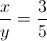Quantity A:Quantity B:The two quantities are equal

Quantity B is greater

Quantity A is greater

The relationship cannot be determined from the information given

The relationship cannot be determined from the information given

Explanation:

Although it seems as though "Quantity B is greater" is the correct answer at first glance, a further analysis indicates that this answer is a trap. Ifandare negative numbers, such asand, thenwould be the larger number. Similarly,is larger if bothandare positive numbers. Thus, it cannot be determined which variable is larger simply based on the information given.

### Example Question #3 : How To Find Proportion

Jane has a collection of coins consisting of pennies, nickels, and dimes in the ratio 6:3:5.

If there are 42 coins in total, how many pennies are in the collection?

15

7

12

9

18

18

Explanation:

First count the total number of parts in the ratio.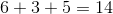Then we can set up a proportion representing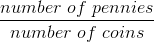As the initial ratio shows, there are 6 pennies for every 14 total coins. In the total set, we have X pennies and 42 total coins. Plugging these numbers into the proportion gives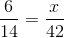.

Finally, we multiply both sides times 42 to isolate x.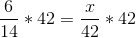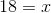← Previous 1

Tired of practice problems?

Try live online GRE prep today.